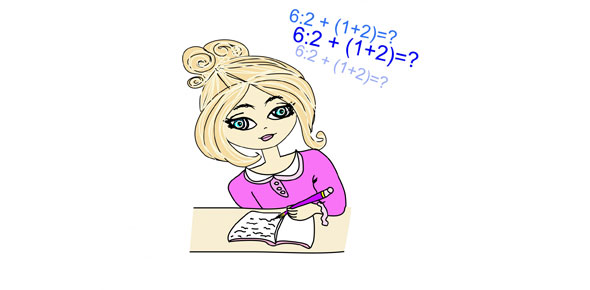SAT Combined Mathematics I

3 QuestionsSettingsThis is an SAT math quiz.

• 1.
If f(x)= (x+[9/pi]), then what does f(4) equal? Round to the nearest whole number.
• A.

7

• B.

10

• C.

3.14

• D.

Infinity

• 2.
Check all that can apply:(x+1)/(x-1) = [1, 3]
• A.

4

• B.

3

• C.

2

• D.

1

• E.

0

• 3.
True or false?10 fits into the set of [3^2, 5^3/2].
• A.

T

• B.

F

Related Topics